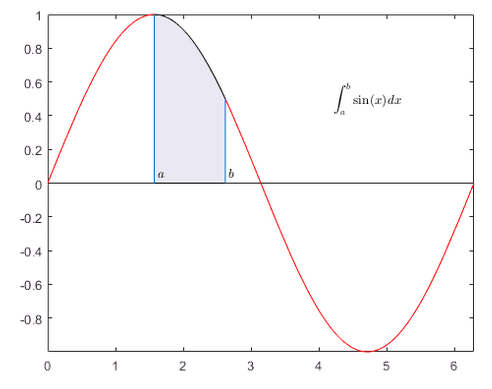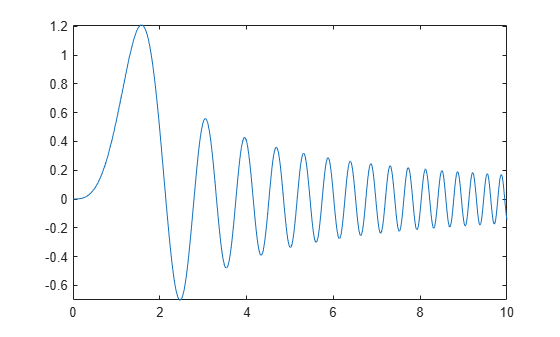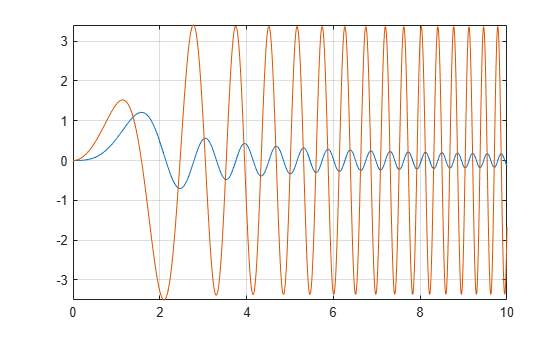Documentation

Integration

This example shows how to compute definite integrals using Symbolic Math Toolbox™.Definite Integral

Show that the definite integral $\underset{a}{\overset{b}{\int }}f\left(x\right)dx$ for $f\left(x\right)=sin\left(x\right)$ on $\left[\frac{\pi }{2},\frac{3\pi }{2}\right]$ is 0.

syms x
int(sin(x),pi/2,3*pi/2)
ans = $0$

Definite Integrals in Maxima and Minima

To maximize $F\left(a\right)={\int }_{-a}^{a}\mathrm{sin}\left(ax\right)\mathrm{sin}\left(x/a\right)\phantom{\rule{0.16666666666666666em}{0ex}}dx$ for $a\ge 0$, first, define the symbolic variables and assume that $a\ge 0$:

syms a x
assume(a >= 0);

Then, define the function to maximize:

F = int(sin(a*x)*sin(x/a),x,-a,a)
F =

Note the special case here for $a=1$. To make computations easier, use assumeAlso to ignore this possibility (and later check that $a=1$ is not the maximum):

assumeAlso(a ~= 1);
F = int(sin(a*x)*sin(x/a),x,-a,a)
F =

$\frac{2 a \left(\mathrm{sin}\left({a}^{2}\right) \mathrm{cos}\left(1\right)-{a}^{2} \mathrm{cos}\left({a}^{2}\right) \mathrm{sin}\left(1\right)\right)}{{a}^{4}-1}$

Create a plot of $F$ to check its shape:

fplot(F,[0 10])Use diff to find the derivative of $F$ with respect to $a$:

Fa = diff(F,a)
Fa =

The zeros of $Fa$ are the local extrema of $F$:

hold on
fplot(Fa,[0 10])
grid onThe maximum is between 1 and 2. Use vpasolve to find an approximation of the zero of $Fa$ in this interval:

a_max = vpasolve(Fa,a,[1,2])
a_max = $1.5782881585233198075558845180583$

Use subs to get the maximal value of the integral:

F_max = subs(F,a,a_max)
F_max = $0.36730152527504169588661811770092 \mathrm{cos}\left(1\right)+1.2020566879911789986062956284113 \mathrm{sin}\left(1\right)$

The result still contains exact numbers $\mathrm{sin}\left(1\right)$ and $\mathrm{cos}\left(1\right)$. Use vpa to replace these by numerical approximations:

vpa(F_max)
ans = $1.2099496860938456039155811226054$

Check that the excluded case $a=1$ does not result in a larger value:

vpa(int(sin(x)*sin(x),x,-1,1))
ans = $0.54535128658715915230199006704413$

Multiple Integration

Numerical integration over higher dimensional areas has special functions:

integral2(@(x,y) x.^2-y.^2,0,1,0,1)
ans = 0

There are no such special functions for higher-dimensional symbolic integration. Use nested one-dimensional integrals instead:

syms x y
int(int(x^2-y^2,y,0,1),x,0,1)
ans = $0$

Line Integrals

Define a vector field F in 3D space:

syms x y z
F(x,y,z) = [x^2*y*z, x*y, 2*y*z];

Next, define a curve:

syms t
ux(t) = sin(t);
uy(t) = t^2-t;
uz(t) = t;

The line integral of F along the curve u is defined as $\int f\cdot du=\int f\left(ux\left(t\right),uy\left(t\right),uz\left(t\right)\right)\cdot \frac{du}{dt}\phantom{\rule{0.16666666666666666em}{0ex}}dt$, where the $\cdot$ on the right-hand-side denotes a scalar product.

Use this definition to compute the line integral for $t$ from $\left[0,1\right]$

F_int = int(F(ux,uy,uz)*diff([ux;uy;uz],t),t,0,1)
F_int =

$\frac{19 \mathrm{cos}\left(1\right)}{4}-\frac{\mathrm{cos}\left(3\right)}{108}-12 \mathrm{sin}\left(1\right)+\frac{\mathrm{sin}\left(3\right)}{27}+\frac{395}{54}$

Get a numerical approximation of this exact result:

vpa(F_int)
ans = $-0.20200778585035447453044423341349$

Mathematical Modeling with Symbolic Math Toolbox

Get examples and videos